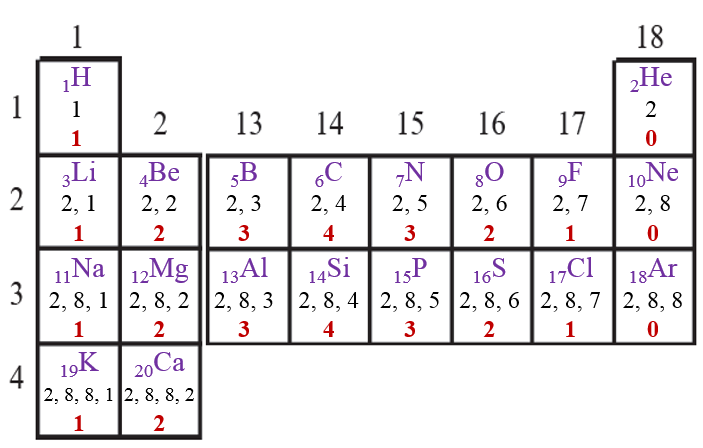###### Watch Video (30:41):
 We can say that the elements with the same number of shells occupied by electrons belong to the same period. The elements in the second period, namely, Li, Be, B, C, N, O, F and Ne have electrons in the two shells, K and L. The elements in the third period, namely, Na, Mg, Al, Si, P, S, Cl and Ar have electrons in the three shells; K, L and M. Write down the electronic configuration of these elements and confirm. In the modern periodic table, electrons are filled in the same shell while going along a period from left to right, and at the begining of the next period a new electron shell starts filling up (See the table 2.8). The number of elements in the first three periods is determined by the electron capacity of the shells and the law of electron octet. (See the Table 2.9)
1. What are the values of ‘n’ for the shells K, L and M?
→ The values of 'n' for the shells K, L and M are 1, 2 and 3 respectively.
1 Mark
2. What is the maximum number of electrons that can be accommodated in a shell? Write the formula
→ The maximum number of electrons that can be accommodated in a shell is given by 2n2. (Where, n = orbit no
1 Mark
3. Deduce the maximum electron capacity of the shells K, L and M.

⇒ For 'K' shell, n = 1.
∴ 2n2 = 2 × 12 = 2 × 1 = 2

⇒ For 'M' shell, n = 2.
∴ 2n2 = 2 × 22 = 2 × 4 = 8

⇒ For 'N' shell, n = 3.
∴ 2n2 = 2 × 32 = 2 × 9 = 18

2 Marks
Periodic trends in the modern periodic table As per the electron holding capacity of shells 2 elements are present in the first period and 8 elements in the second period. The third period also contains only eight elements due to the law of electron octet. There are few more factors which control the filling of electrons in the subsequent periods which will be considered in the next standards. The chemical reactivity of an element is determined by the number of valence electrons in it and the shell number of the valence shell. The information on these points is obtained from the position of the element in the periodic table. That is, the modern periodic table has proved useful for study of elements. When the properties of elements in a period or a group of the modern periodic table are compared, certain regularity is observed in their variations. It is called the periodic trends in the modern periodic table. In this standard we are going to consider the periodic trends in only three properties of elements; namely, valency, atomic size and metallic- nonmetallic character. Valency : You have learnt in the previous standard that the valency of an element is determined by the number of electrons present in the outermost shell of its atoms, that is, the valence electrons.
1. What is the relationship between the electronic configuration of an element and its valency?
→ The valency of an element is determined by the number of electrons in the outermost shell.
2. The atomic number of beryllium is 4 while that of oxygen is 8. Write down the electronic configuration of the two and deduce their valency from the same.
→ The electronic configurations are given below:
4Be: 2, 2 and 8O: 2, 6
Beryllium can donate two electrons and become stable while Oxygen can accept two electrons and become stable.
∴ The valency of both of them is 2.
3. The table on the next page [Page No. 25] is made on the basis of the modern periodic table. Write in it the electronic configuartion of the first 20 elements below the symbol, and write the valency (as shown in a separate box).
→ The completed table is given below:4. What is the periodic trend in the variation of valency while going from left to right within a period? Explain your answer with reference to period 2 and period 3.
→ As we move from left to right in a period, initially the valency goes on increasing from 1 to 4. Thereafter it goes on decreasing and finally becomes 0.
5. What is the periodic trend in the variation of valency while going down a group? Explain your answer with reference to the group 1, group 2 and group 18.
→ As we move from down in a group, the valency remains the same. The valency for group 1 elements is 1, group 2 elements is 2 and group 18 elements is 0.